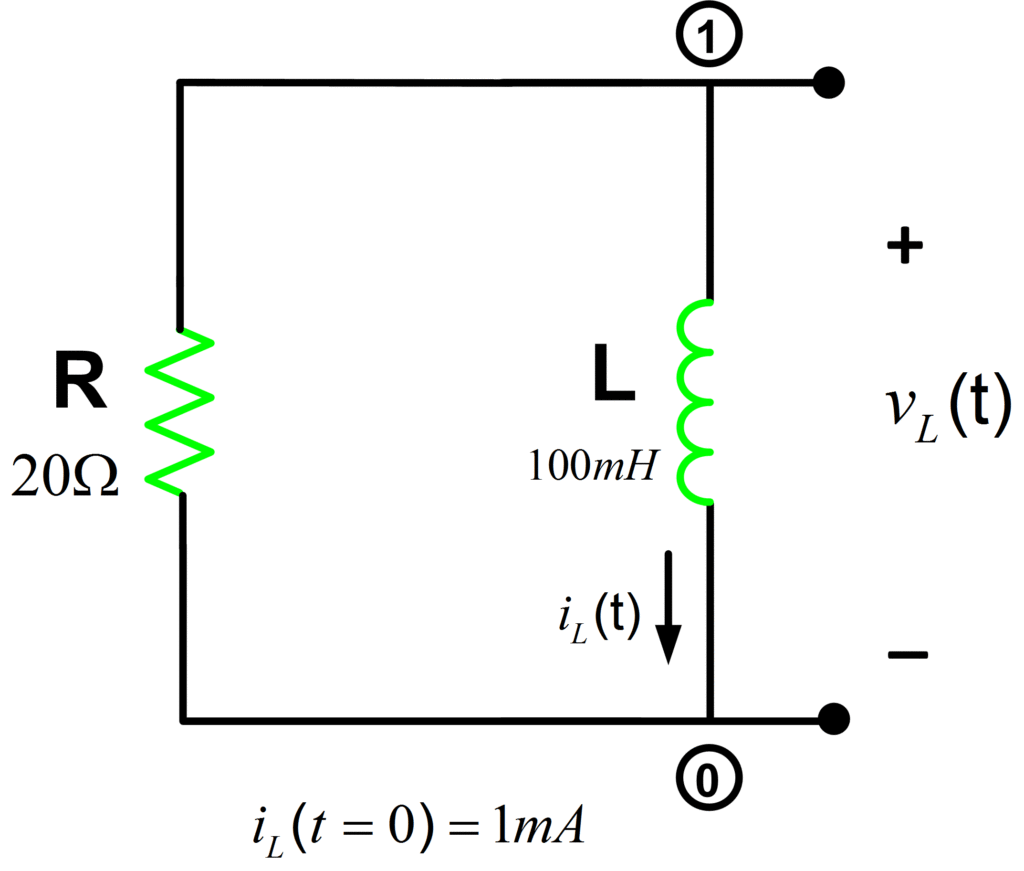Home / Circuits with Matlab / RL Circuit Analysis using Matlab

# RL Circuit Analysis using Matlab

Want create site? Find Free WordPress Themes and plugins.

Determine the voltage across the inductor in an RL circuit:Let us compute the voltage across the inductor for t≥0 using the following expression:

${{v}_{L}}(t)=-R{{I}_{lo}}{{e}^{-t/\tau }}u(t)$

Whereas the inductor initial current is 1mA and time constant τ=L/R=5ms.

It’s time to write some code in Matlab to calculate the inductor voltage:

%RL Circuit Analysis
clear all;close all;clc
%%Circuit Parameters
R= 20; % Resistance (20 Ohm)
L= 100e-3; % Inductance (100mH)
tau=L/R; % RL Time Constant
I_lo=1e-3; % Inductor Initial Current
Time=0:tau/100:5*tau; % Sampling Time
V_L=-R*I_lo.*exp(-Time./tau).*heaviside(Time);
plot(Time,V_L)
xlabel('Time (s)')
ylabel('Amplitude (V)')
title('V_L')
axis([0 5*tau -21e-3 0]) % Manual Axis Limits adjustment
%=============================================


Results:Did you find apk for android? You can find new Free Android Games and apps.